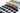Mar 04, 2019

How to find out the amount of debt on the loan: 3 easy ways

When we take a loan (secured or otherwise), the total monthly commitment to the bank payable is broken up into two components. The principal sum repayable and the interest (or debt) component payable. It is important to know the interest (or debt) payable based on the type of loan accepted. The different category of loans will have different interest rate percentages.

Usually, tangible investments such as automobiles and property will have a lower interest rate as compared to a personal, credit card or a payday loan.

Usually during the beginning of the tenure of a loan, the interest (or debt) component contributes to a higher percentage than the repayable principal sum amount.

One method of calculating the interest (or debt) is by multiplying the principal with the interest rate by the time period. Once the interest is calculated, it is usually recorded as an accrued liability by the borrower. This is a simple paper napkin method to be used to calculate the whole interest (or debt) amount without serious consideration of the percentage change in the interest (or debt) ratio to the principal sum.

Another manual way to calculate the interest of a 1 (one) year loan is to divide multiply the assigned interest rate by the principal sum. This method will not be suitable for multiple year loans. This is a rudimentary interest (or debt) calculation to assess the interest (or debt) amount for short-term borrowings.

The easiest alternative method is to use a financial loan calculator which is available for use online. Most reputable finance and accounting firms have a standardized loan calculator. Its complex algorithm is capable to distinguish between the percentage change of the interest (or debt) amount from its principal from the first installment, to its last.

Mar 04, 2019